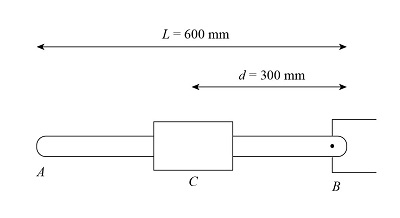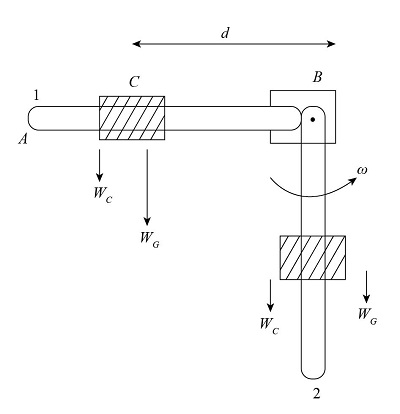# A collar with a mass of 1 kg is rigidly attached at a distance d = 300 mm from the end of a...

## Question:

A collar with a mass of 1 kg is rigidly attached at a distance d = 300 mm from the end of a uniform slender rod AB. The rod has a mass of 3 kg and is of length L = 600 mm. Knowing that the rod is released from rest, determine the angular velocity of the rod after it has rotated through 90 degrees.

## Energy conservation:

The basic idea behind the conservation of energy is that the complete depletion of energy is not possible, rather it gets converted from one form to the other in ongoing processes. For example: Throwing a ball from a certain height converts potential energy to kinetic energy of the ball.

Given data:

• Mass of collar is, {eq}{m_c} = 1\;{\rm{kg}} {/eq}.
• Mass of rod is, {eq}{m_r} = 3\;{\rm{kg}} {/eq}.
• distance of collar is, {eq}d = 300\;{\rm{mm}} {/eq}.

The figure for the slender rod is shown below,The rod rotated at 90 degrees is shown as,If {eq}{W_C}\;{\rm{and}}\;{W_R} {/eq} are the weight of the collar and the rod respectively.

Taking position 1 as datum the sum of kinetic energy and potential energy is zero.

{eq}K{E_1} + P{E_1} = 0 {/eq}

The potential energy at position 2 as,

{eq}P{E_2} = - {W_C}d - {W_R}\dfrac{L}{2} {/eq}

The kinetic energy at position 2 is,

{eq}\begin{align*} K{E_2} &= \dfrac{1}{2}{m_R}v_R^2 + \dfrac{1}{2}{I_R}{\omega ^2} + \dfrac{1}{2}{m_C}v_C^2\\ K{E_2} &= \dfrac{1}{2}{m_R}{\left( {\dfrac{L}{2}\omega } \right)^2} + \dfrac{1}{2}{I_R}\left( {\dfrac{1}{{12}}{m_R}{L^2}} \right){\omega ^2} + \dfrac{1}{2}{m_C}{d^2}{\omega ^2}\\ K{E_2} &= \dfrac{1}{6}{m_R}{L^2}{\omega ^2} + \dfrac{1}{6}{m_C}{d^2}{\omega ^2} \end{align*} {/eq}

Apply laws of conservation of energy as,

{eq}\begin{align*} K{E_1} + P{E_1} &= K{E_2} + P{E_2}\\ 0 &= \dfrac{1}{6}{m_R}{L^2}{\omega ^2} + \dfrac{1}{2}{m_C}{d^2}{\omega ^2} - {W_C}d - {W_R}\dfrac{L}{2}\\ {\omega ^2}\left( {\dfrac{1}{6}{m_R}{L^2} + \dfrac{1}{2}{m_C}{d^2}} \right) &= {W_C}d + {W_R}\dfrac{L}{2}\\ {\omega ^2}\left( {\dfrac{1}{6}{m_R}{L^2} + \dfrac{1}{2}{m_C}{d^2}} \right) &= {m_C}gd + {m_R}g\dfrac{L}{2} \end{align*} {/eq}

Solve by substituting the value in the above expression as,

{eq}\begin{align*} {\omega ^2}\left( {\dfrac{1}{6} \times 3 \times {{0.6}^2} + \dfrac{1}{2} \times 1 \times {{0.3}^2}} \right) &= 1 \times 9.81 \times 0.3 + 3 \times 9.81 \times \dfrac{{0.6}}{2}\\ {\omega ^2}\left( {0.225} \right) &= 11.772\\ \omega &= \sqrt {52.32} \\ \omega &= 7.233\;{{{\rm{rad}}} {\left/ {\vphantom {{{\rm{rad}}} {\rm{s}}}} \right. } {\rm{s}}} \end{align*} {/eq}

Thus, the angular velocity of the rod is, {eq}7.233\;{{{\rm{rad}}} {\left/ {\vphantom {{{\rm{rad}}} {\rm{s}}}} \right. } {\rm{s}}} {/eq}.